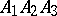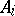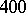# Triangle centre

(diff) ← Older revision | Latest revision (diff) | Newer revision → (diff)

Given a triangle, a triangle centre is a point dependent on the three vertices of the triangle in a symmetric way. Classical examples are:

the centroid (i.e. the centre of mass), the common intersection point of the three medians (see Median (of a triangle));

the incentre, the common intersection point of the three bisectrices (see Bisectrix) and hence the centre of the incircle (see Plane trigonometry);

the circumcentre, the centre of the circumcircle (see Plane trigonometry);

the orthocentre, the common intersection point of the three altitude lines (see Plane trigonometry);

the Gergonne point, the common intersection point of the lines joining the vertices with the opposite tangent points of the incircle;

the Fermat point (also called the Torricelli point or first isogonic centre), the pointthat minimizes the sum of the distances;

the Grebe point (also called the Lemoine point or symmedean point), the common intersection point of the three symmedeans (the symmedean throughis the isogonal line of the median through, see Isogonal);

the Nagel point, the common intersection point of the lines joining the vertices with the centre points of the corresponding excircles (see Plane trigonometry).

In [a1],different triangle centres are described.

The Nagel point is the isotomic conjugate of the Gergonne point, and the symmedean point is the isogonal conjugate of the centroid (see Isogonal for both notions of "conjugacy" ).